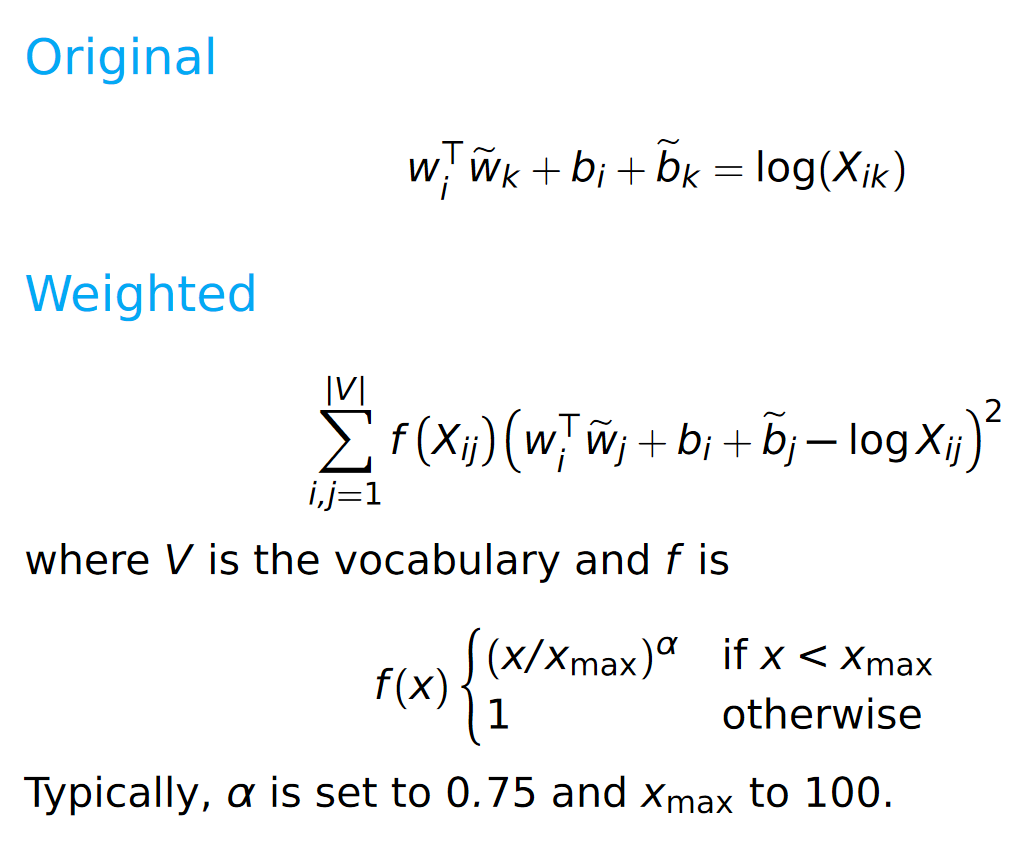Vector Space Models

# Distributed word representations #

## Meaning representations #

Co-occurrence matrix: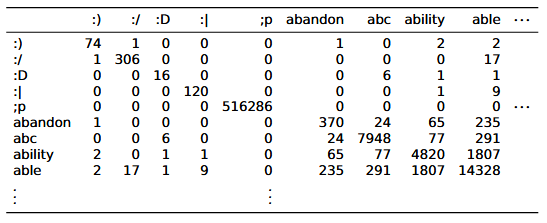Meaning can be present in such a matrix.

• If a word co-occurs often with “excellent,” it likely is a positive word; if it co-occurs often with “terrible,” it likely denotes something negative

## Guiding hypothesis for vector-space models #

The meaning of a word is derived from its use in a language. If two words have similar vectors in a co-occurrence matrix, they tend to have similar meanings (Turney and Pantel, 2010).

## Feature representations of data #

• the movie was horrible becomes [4, 0, 0.25] (4 words, 0 proper names, 0.25 concentration of negative words)
• Reduces noisy data to restricted feature set

## What do we define co-occurrence as? #

For a sentence, e.g. from swerve of shore to bend of bay, brings

Consider the word “to”

• Window: how many words around “to” (in both directions) do we want to focus on?
• Scaling: how to weight words in the window?
• Flat: treat everything equally
• Inverse: word is weighted 1/n if it is distance n from the target word

Larger, flatter windows capture more semantic information, whereas smaller, more scaled windows capture more syntactic information

Can also consider different unit sizes - words, sentences, etc

## Constructing data #

• Tokenization
• Annotation
• Tagging
• Parsing
• Feature selection

## Matrix design #

### word x word #

• Rows and columns represent individual words
• Value `a_ij` in a matrix represents how many times words `i` and `j` co-occur with each other in a given set of documents
• Very dense (lots of nonzero entries)! Density increases with more documents in the corpus
• Dimensionality remains fixed as we bring in new data as long as we pre-decide on vocabulary

### word x document #

• Rows represent words; columns represent documents
• Value `a_ij` in a matrix represents how many times word `i` occurs in document `j`
• Very sparse: may be hard to compute certain operations, but easy storage

### word x discourse context #

• Rows represent words; columns represent discourse context labels
• Labels are assigned by human annotators based on what type of context the sentence is (i.e., acceptance dialogue, rejecting part of previous statement, phrase completion, etc)
• Value `a_ij` in a matrix represents how many times word `i` occurs in discource context `j`

### Other designs #

• word x search proximity
• word x dependency relations

Note: Models like GloVe and word2vec provide packaged solutions that pre-chose from these design choices.

## Vector comparison (similarity) #

Within the context of this example: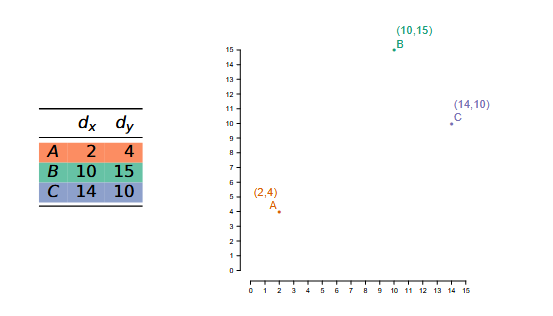Note that B and C are close in distance (frequency info), but A and B have a similar bias (syntactic/semantic info)

### Euclidean #

For vectors `u`, `v` of `n` dimensions:

This measures the straight-line distance between `u` and `v` capturing the pure distance aspect of similarity

Note: Length normalization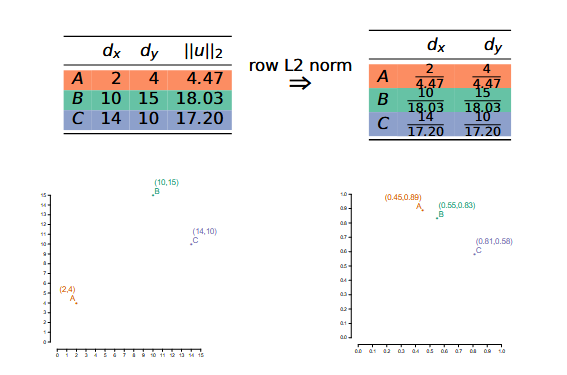This captures the bias aspect of similarity

### Cosine #

For vectors `u`, `v` of `n` dimensions:

• Division by the length effectively normalizes vectors
• Captures the bias aspect of similarity
• Not considered a proper distance metric because it fails the triangle inequality; however, the following does:

• But the correlation between these two metrics is nearly perfect, so in practice, use the simpler one

### Other metrics #

• Matching
• Dice
• Jaccard
• KL (distance between probability distributions)
• Overlap

## Reweighting #

Goal: Amplify important data useful for generalization, because raw counts/frequency are poor proxy for semantic information

### Normalization #

• L2 norming (see above)
• Probability distribution: divide values by sum of all values

### Observed/Expected #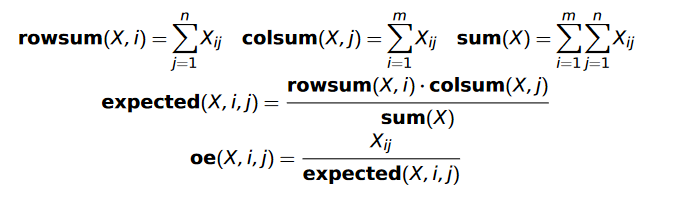Intuition: Keeps words in idioms co-occurring more than expected; other word pairs co-occur less than expected

### Pointwise Mutual Information (PMI) #

This is the log of observed count divided by expected count.

### Positive PMI #

PMI undefined when `X_{ij} = 0`. So:

### TF-IDF #

For a corpus of documents D: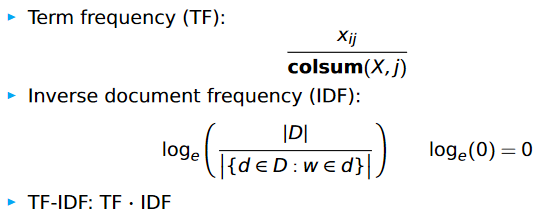## Dimensionality reduction #

### Latent Semantic Analysis #

• Also known as Trucated Singular Value Decomposition (Truncated SVD)
• Standard baseline, difficult to beat

Intuition:

• Fitting a linear model onto data encourages dimensionality reduction (since we can project data onto the model); this captures greatest source of variation in the data
• We can continue adding linear models to capture other sources of variation

Method:

Any matrix of real numbers can be written as

where `S` is a diagonal matrix of singular values and `T` and `D^T` are orthogonal. In NLP, `T` is the term matrix and `D^T` is the document matrix.

Dimensionality reduction comes from being selective about which singular values and terms to include (i.e., capturing only a few sources of variation in the data).

### Autoencoders #

• Flexible class of deep learning architectures for learning reduced dimensional representations

Basic autoencoder model: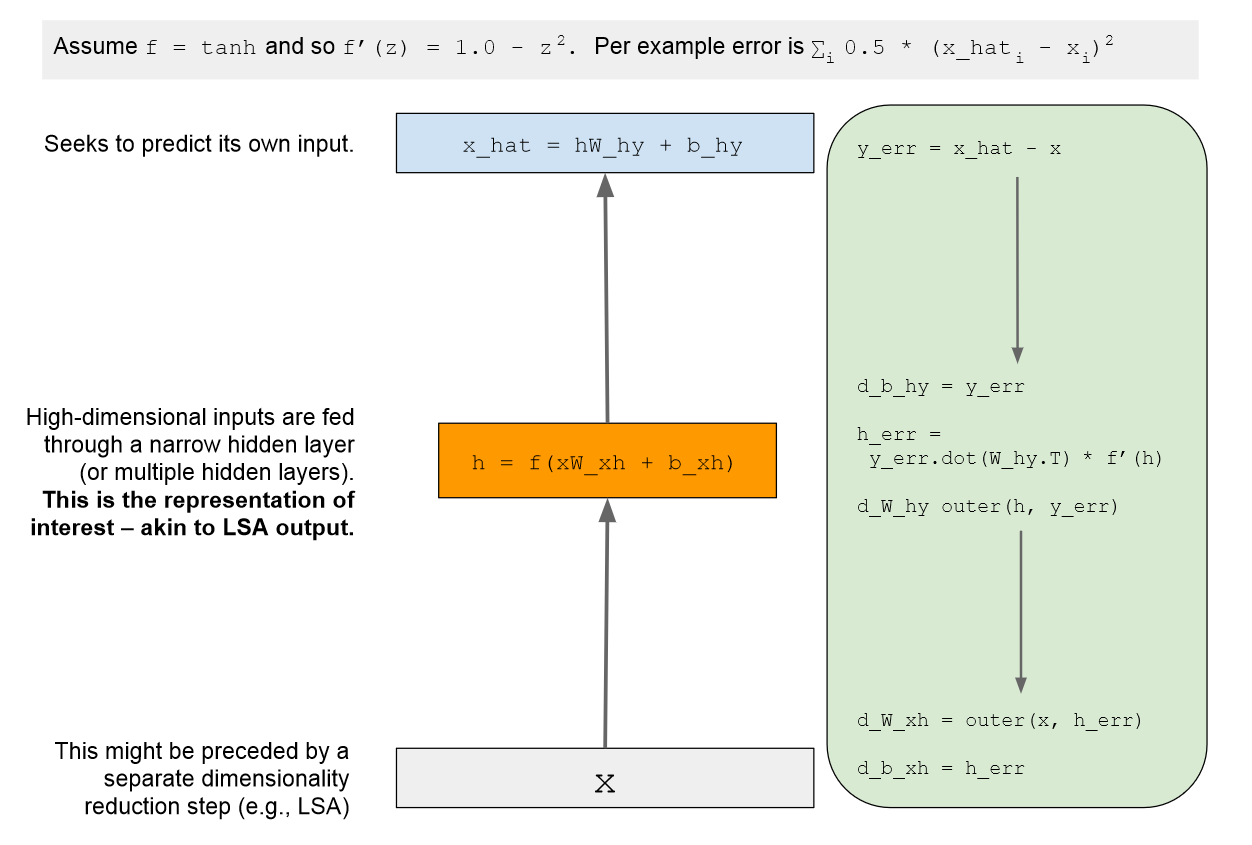### GloVe #

• Goal is to learn vectors for words such that their dot product is proportional to their log probability of co-occurrence